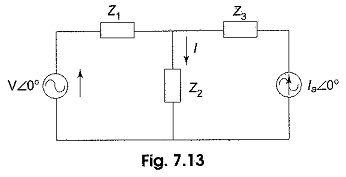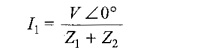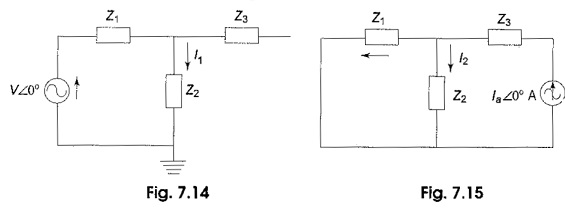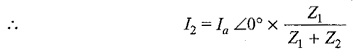## Superposition Theorem Formula:

The Superposition Theorem Formula also can be used to analyses ac circuits containing more than one source. The superposition theorem states that the response in any element in a circuit is the vector sum of the responses that can be expected to flow if each source acts independently of other sources.

As each source is considered, all of the other sources are replaced by their internal impedances, which are mostly short circuits in the case of a voltage source, and open circuits in the case of a current source.

This theorem is valid only for linear systems. In a network containing complex impedance, all quantities must be treated as complex numbers.

Consider a circuit which contains two sources as shown in Fig. 7.13.Now let us find the current I passing through the impedance Z2 in the circuit. According to the superposition theorem, the current due to voltage source V ∠0° V is I1 with current source Ia ∠0° A open circuited.The current due to Ia ∠0° A is I2 with voltage source V ∠0° short circuited.The total current passing through the impedance Z2 is

Scroll to Top